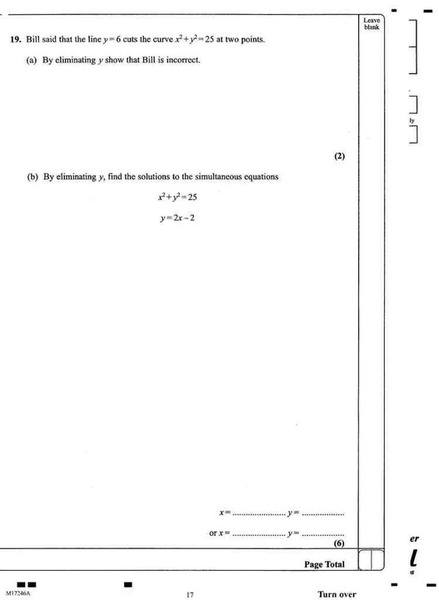# Eliminating yFor (a) I got x = $$\sqrt{-11}$$ which you cant do as you cant square root a negitive number, I cant help but feel im wrong

#### Attachments

cristo
Staff Emeritus
For (a) I got x = $$\sqrt{-11}$$ which you cant do as you cant square root a negitive number, I cant help but feel im wrong

Well, for that reason I would say that Bill is wrong; not you!

and for (b) I began be substituting y =2x - 2 into the y²

x² + (2x-2)(2x-2) = 25

5x² -8x -21 = 0

am I doing the right thing so far. When I used the quadratic formula I got a decimal number, not a whole number and the question doesn't say anything about rounding to a degree of accuracy so I presume the answer is whole numbersarildno
Homework Helper
Gold Member
Dearly Missed
No, you may presume that the answer is to be given exactly in b)!

That is you have the two solutions:
$$x=\frac{-(-8)\pm\sqrt{(-8)^{2}-4*5*(-21)}}{2*5}=\frac{8\pm\sqrt{484}}{10}=\frac{8\pm{22}}{10}$$
As it happens, you get rational solutions here, otherwise, the exact solutions would involve square root symbols explicitly.

cristo
Staff Emeritus
and for (b) I began be substituting y =2x - 2 into the y²

x² + (2x-2)(2x-2) = 25

5x² -8x -21 = 0

am I doing the right thing so far. When I used the quadratic formula I got a decimal number, not a whole number and the question doesn't say anything about rounding to a degree of accuracy so I presume the answer is whole numbersWhat do you get when you solve this quadratic equation? The answer does not necessarily have to be a whole number, especially if it is only a short decimal. The solution to this equation is such that it can easily be written exactly.

HallsofIvy
Homework Helper
Or, to put it another way,
5x2- 8x+ 21= (5x+ 7)(x- 3)= 0

i must of made an error in typing it into my calculator. For one of x's solutions I got somthing like 4.926537173 (i pressed random keys after the 3.s.f). Ill check over my work now...

okay, when typing it in I think I calculated the stuff inside the root wrong

x = -7 or 3
y = -16 or 4

arildno
Homework Helper
Gold Member
Dearly Missed
Eeeh??

never mind, but ive posted the (i think correct answers now above).

However im concerned that -16² + -7² dont equal 25

HallsofIvy
Homework Helper
arildno suggested
$$x=\frac{-(-8)\pm\sqrt{(-8)^{2}-4*5*(-21)}}{2*5}=\frac{8\pm\sqrt{484}}{10}=\frac{8\pm{22 }}{10}$$

and I told you that 5x2- 8x+ 21= (5x+ 7)(x- 3)= 0.

How could you possibly get "x = -7 or 3" from that?

(8 + 22)/10 = 3
(8-22)/10 = 1.4

woops

EDIT: Making y 4 or 4.8

HallsofIvy
Homework Helper
Well, I would have said 7/5 but but I grew up BC (before calculators).

arildno
Homework Helper
Gold Member
Dearly Missed
Actually, I would say -7/5..

$$x=\frac{-(-8)\pm\sqrt{(-8)^{2}-4*5*(-21)}}{2*5}=\frac{8\pm\sqrt{484}}{10}=\frac{8\pm{22 }}{10}$$

8+22 = 30/10 = 3
8-22 = -14/10 = -1.4

feed that into the equation y = 2(x) - 2

2(3) - 2 = 6- 2 = 4
2(-1.4) - 2 = -2.8-2 = -4.8

I would think that must be right?

HallsofIvy